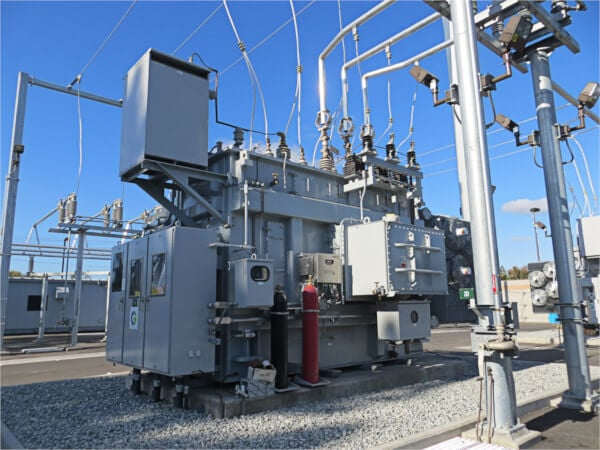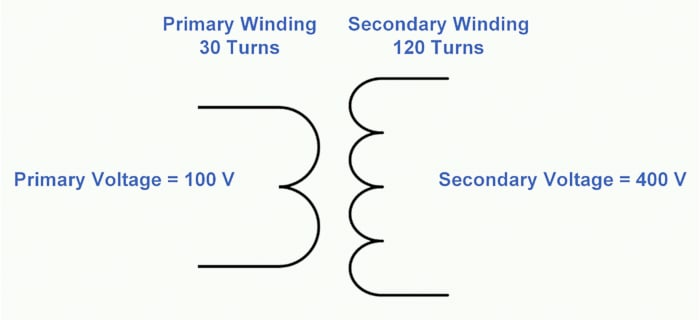Technical Article

# Calculating Transformer Voltage

September 19, 2023 by Ahmed Sheikh

## This article presents three fundamental methods for calculating transformer voltages using the voltage-to-turns formula, turns ratios, and volts per turn, and also examines the differences between ideal and real transformer turns ratios.

First, a little terminology.

The coil of the transformer with a voltage applied to it is always called the primary coil or primary winding, and the voltage applied is always called the primary voltage (VP). The coil that has a voltage induced in it by the magnetic field of the primary winding is always called the secondary coil, or secondary winding, and the voltage induced in it is called the secondary voltage (VS). If we know the number of turns on the primary and secondary windings, we can calculate the value of one of the voltages if the other voltage is known.### Solving for Transformer Voltages Using the Voltage-to-Turns Formula

What would the secondary voltage be if a transformer were supplied with 120 volts to a primary winding with 60 turns and the secondary winding had 15 turns?

The rule is:

$\frac{V_{P}}{V_{S}}=\frac{Primary\,Turns}{Secondary\,Turns}$

If we put in the numbers we know, we have:

$\frac{120V_{p}}{V_{S}}=\frac{60\,Primary\,Turns}{15\,Secondary\,Turns}$

Cross-multiplying to solve for VS, we get 60 × VS = 120 × 15.

Simplifying the equation gives us the following:

$V_{S}=\frac{120\,15}{60}=30V$

Therefore, the secondary voltage is 30 volts.##### Figure 1. Transformer secondary voltage calculation. Image used courtesy of Ahmed Sheikh

Here is another example. This time, a transformer has a primary winding with 30 turns and a secondary winding with 120 turns. If the secondary voltage is 400 volts, what is the primary voltage?

We put the values we know into the first rule for transformers, which says that the ratio of turns is equal to the ratio of voltages:

$\frac{V_{p}}{400V_{S}}=\frac{30\,Primary\,Turns}{120\,Secondary\,Turns}$

Cross-multiplying to solve for VP gives us 120 × VP = 30 × 400.

Dividing both sides by 120 turns to solve for VP gives us:

VP = 100 V### Solving for Transformer Voltages Using Turn Ratios

Another way to think of this last problem is that the ratio of primary turns to secondary turns is 1 to 4. As we have seen, the ratio of primary voltage to secondary voltage is the same as that of primary turns to secondary turns, so the voltage ratio must also be 1 to 4. Therefore, the primary voltage must be one-fourth greater than the secondary voltage. If the secondary voltage is 400 volts, the primary voltage is:

$\frac{4000V_{S}}{4}=\,100\,V$

Here is another example. What is the secondary voltage of a transformer if the ratio of primary turns to secondary turns is 6 to 1 and the primary voltage is 48 volts?

Because the number of turns on the primary is 6 times larger than the number of turns on the secondary, we can say that the primary voltage is 6 times larger than the secondary voltage. Thus, the turns on the secondary are one-sixth as many as the turns on the primary, so the secondary voltage is one-sixth of the primary voltage:

$\frac{48V_{p}}{6}=8V$

The standard way of expressing transformer ratios is to use the primary voltage first and the secondary voltage second. In our example, the ratio is 6 to 1. A transformer with a primary voltage of 120 volts and a secondary voltage of 240 would have a 1 to 2 ratio.

### Solving for Transformer Voltages Using Volts Per Turn

We can also solve for transformer voltage by using the volts per turn on each winding. If there are 120 turns on the secondary winding and the induced voltage is 600 volts, 5 volts per turn (600 volts ÷ 120 turns) are induced in the secondary winding. Since the primary winding has almost the same volts per turn induced, if the primary coil had 30 turns, the primary voltage would be 150 volts (30 turns × 5 volts per turn). Does this add up? The primary winding has fewer turns, so the primary voltage should be smaller. How much smaller? The ratio of turns is 30 turns pri to 120 turns sec or 1 to 4, so the primary voltage should be one-fourth of the secondary voltage, or 150 volts.

Here is another example. The transformer must supply 24 volts to a load. The supply voltage is 480 volts, and the number of turns on the primary winding is 1,200. What is the number of turns on the secondary winding?

The volts per turn on the primary winding are:

$\frac{400Volts}{1200\,Primary\,Turns}=0.4Volts\,per\,Turns$

The secondary winding turns have the same volts per turn induced on them. Because each turn of the secondary has .4 volts induced in it, to induce 24 volts, there must be:

$\frac{24Volts}{0.4Volts\,per\,Turns}=60\,Secondary\,Turns$

In solving any ratio problem, we can always calculate the fourth value if we know three of the values. Look at the last example: A transformer must supply 24 volts from a 480-volt supply, and the number of turns on the primary is 1,200, so to find the secondary turns required, we fill in the information we know in the transformer ratio:

$\frac{480V}{24V}=\frac{1200\,Primary\,Turns}{Secondary\,Turns}$

Cross-multiplying to solve for turns sec gives us the following:

480 V × Secondary Turns = 24 V × 1200 Primary Turns

Dividing both sides by 480 volts to solve for secondary turns gives us the following:

Secondary Turns = 60 Turns

The secondary voltage is smaller, so the number of secondary turns should be less. The ratio of VP to VS is 20 to 1, so the ratio of turns should also be 20 to 1: 60 × 20 = 1,200; this is the number of turns on the primary, so our answer is correct.### Ideal Transformer vs. Real Transformer Turns Ratios

The ratios we are using are for an ideal transformer – one that does not have any losses. Real transformers have losses and must compensate for these losses by slightly changing the ratio. However, whenever a voltage rating is applied to a transformer's primary and secondary coils, we can think of the coil turn ratios as the same as the voltage ratios without being incorrect. For example, suppose a transformer has a primary winding with a voltage rating of 240 volts and a secondary winding with 24 volts. In that case, we can safely say that if we apply 100 volts to the primary coil, there will be a secondary voltage very close to 10 volts because the ratio of turns is 10 to 1. In reality, we rarely know the actual number of turns on either the primary or secondary winding. However, we can be sure that whatever the number of turns is, the ratio between the turns in each winding is about the same as that of the voltages.

1 Comment